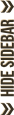With cordite in the air, splintered steel, shell casings and powder burns, there’s only one explanation...
If you have any trouble with SG, this is the place to ask.

Moderator: Pardner

### [Tutorial] Lag every minute ?

Introduction

Do you use the Wi-Fi technology to have internet?
Do you have Windows XP?
Do you have a ping at around 1000 milliseconds every one or two minutes?
This is annoying when you have a stick of TNT near to blow in your hand, isn't it?I had the same problem a long time ago and this is now the time to share to the online community my solution of this problem.
Before creating this small tutorial, I needed to know that it was working not only on my own computer, this was done recently.

Contents
• Spotting the problem
• Theory
• Let's solve it

Spotting the problem

As I already said, this problem occures on computer which are running Windows XP and are having internet with the Wi-Fi.
You can see the problem while playing with the sudden high ping you have every one or two minutes, just count to be sure.
You actually don't see the problem while just surfing on the net because this sudden high ping is too short to be seen on a website. This is another problem while playing online because you actually need a regular connection. Here is some ping statistic I did:
Code: Select all
`\$ ping -t www.google.comEnvoi d'une requête 'ping' sur www.l.google.com [74.125.39.106] avec 32 octets de données:Réponse de 74.125.39.106: octets=32 temps=26 ms TTL=52Réponse de 74.125.39.106: octets=32 temps=28 ms TTL=52Réponse de 74.125.39.106: octets=32 temps=28 ms TTL=52Réponse de 74.125.39.106: octets=32 temps=26 ms TTL=52Réponse de 74.125.39.106: octets=32 temps=28 ms TTL=52Réponse de 74.125.39.106: octets=32 temps=28 ms TTL=52Réponse de 74.125.39.106: octets=32 temps=28 ms TTL=52Réponse de 74.125.39.106: octets=32 temps=28 ms TTL=52Réponse de 74.125.39.106: octets=32 temps=28 ms TTL=52Réponse de 74.125.39.106: octets=32 temps=29 ms TTL=52Réponse de 74.125.39.106: octets=32 temps=28 ms TTL=52Réponse de 74.125.39.106: octets=32 temps=29 ms TTL=52Réponse de 74.125.39.106: octets=32 temps=27 ms TTL=52Réponse de 74.125.39.106: octets=32 temps=28 ms TTL=52Réponse de 74.125.39.106: octets=32 temps=28 ms TTL=52Réponse de 74.125.39.106: octets=32 temps=27 ms TTL=52Réponse de 74.125.39.106: octets=32 temps=26 ms TTL=52Réponse de 74.125.39.106: octets=32 temps=28 ms TTL=52Réponse de 74.125.39.106: octets=32 temps=28 ms TTL=52Réponse de 74.125.39.106: octets=32 temps=28 ms TTL=52Réponse de 74.125.39.106: octets=32 temps=26 ms TTL=52Réponse de 74.125.39.106: octets=32 temps=28 ms TTL=52Réponse de 74.125.39.106: octets=32 temps=28 ms TTL=52Réponse de 74.125.39.106: octets=32 temps=28 ms TTL=52Réponse de 74.125.39.106: octets=32 temps=26 ms TTL=52Réponse de 74.125.39.106: octets=32 temps=26 ms TTL=52Réponse de 74.125.39.106: octets=32 temps=28 ms TTL=52Réponse de 74.125.39.106: octets=32 temps=29 ms TTL=52Réponse de 74.125.39.106: octets=32 temps=28 ms TTL=52Réponse de 74.125.39.106: octets=32 temps=27 ms TTL=52Réponse de 74.125.39.106: octets=32 temps=27 ms TTL=52Réponse de 74.125.39.106: octets=32 temps=1833 ms TTL=52Réponse de 74.125.39.106: octets=32 temps=27 ms TTL=52Réponse de 74.125.39.106: octets=32 temps=26 ms TTL=52Réponse de 74.125.39.106: octets=32 temps=27 ms TTL=52Réponse de 74.125.39.106: octets=32 temps=27 ms TTL=52Réponse de 74.125.39.106: octets=32 temps=27 ms TTL=52Réponse de 74.125.39.106: octets=32 temps=27 ms TTL=52Réponse de 74.125.39.106: octets=32 temps=29 ms TTL=52Réponse de 74.125.39.106: octets=32 temps=27 ms TTL=52Réponse de 74.125.39.106: octets=32 temps=27 ms TTL=52Réponse de 74.125.39.106: octets=32 temps=27 ms TTL=52`

You can clearly see the spot where I get a sudden high ping, 1833 milliseconds here, even if my computer is in frenchTheory

This is the fault of Windows XP here. The computer is actually scanning every new Wi-Fi hotspots every one or two minutes. This is the reason that you lost your internet connection in that time. This task on Windows is called the "Windows Zero Configuration SerViCe", let's just call it WZCSVC, okay?
The aim now is to disable this task without being disconnected from the current Wi-Fi hotspot.

Let's solve it

This is finally a simple way to get rid of this problem, just disable that task at the good moment and you're done.

The complete way:

1) Create one text file. (.txt)
2) Open it and add into it the following text:
Code: Select all
`@echo offnet stop WZCSVC@echo on`

3) save it and rename it as WZCSVC_off.bat . You might activate the possibility to show the extension of files to be able to do that step.

This is the file which will disable the WZCSVC. You just need, after being connected to your Wi-Fi, run that script by double clicking on it. You will need to do that on every computer's boot.

If you loose your connection to your Wi-Fi hotspot, you won't be able to reconnect so you need also a script that re-enable that task. Just change the step 2) with that following code:
Code: Select all
`@echo offnet start WZCSVC@echo on`

and save it in another file named WZCSVC_on.bat .

The fast and lame way:

Conclusion

I hope you solved the high ping issue with that small trick, it worked on my computer. This was just a small configuration's problem which was solved easily. If you have other problem, don't be scared to reply or send me some PM. I have tested that way only on Windows XP, having an answer on the other system would be nice.
I apologize also with my bad english, i won't be angry if I someone tells me I made some mistakes, I will just correct them.

Source

- http://jelnet.free.fr/jlnt_indx.htm which explains the trick with the script, and some other (french)
"Chuck Norris had to shorten his beard in the presence of Richard Stallman because two beards that awesome, so close would segfault the universe (again)."Barto

Posts: 356
Joined: Fri Oct 23, 2009 5:08 pm
Location: Switzerland